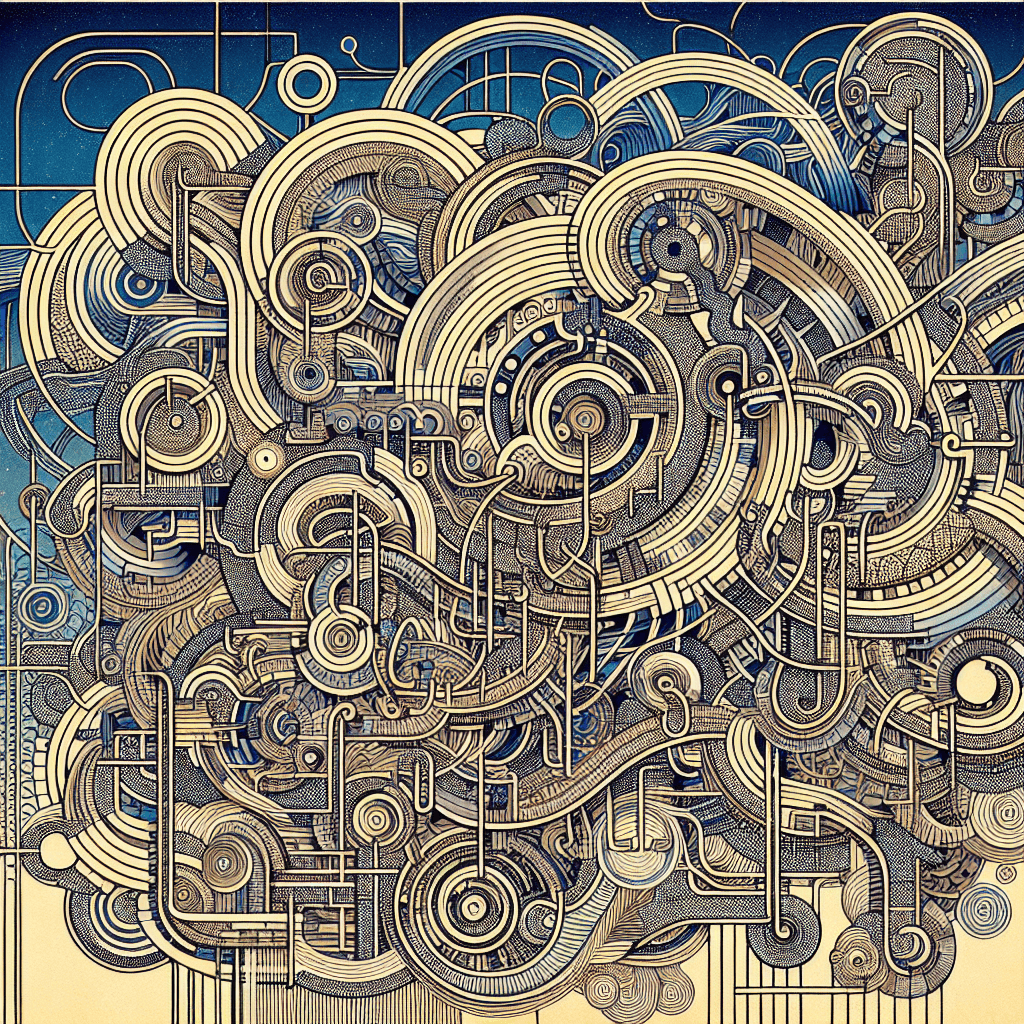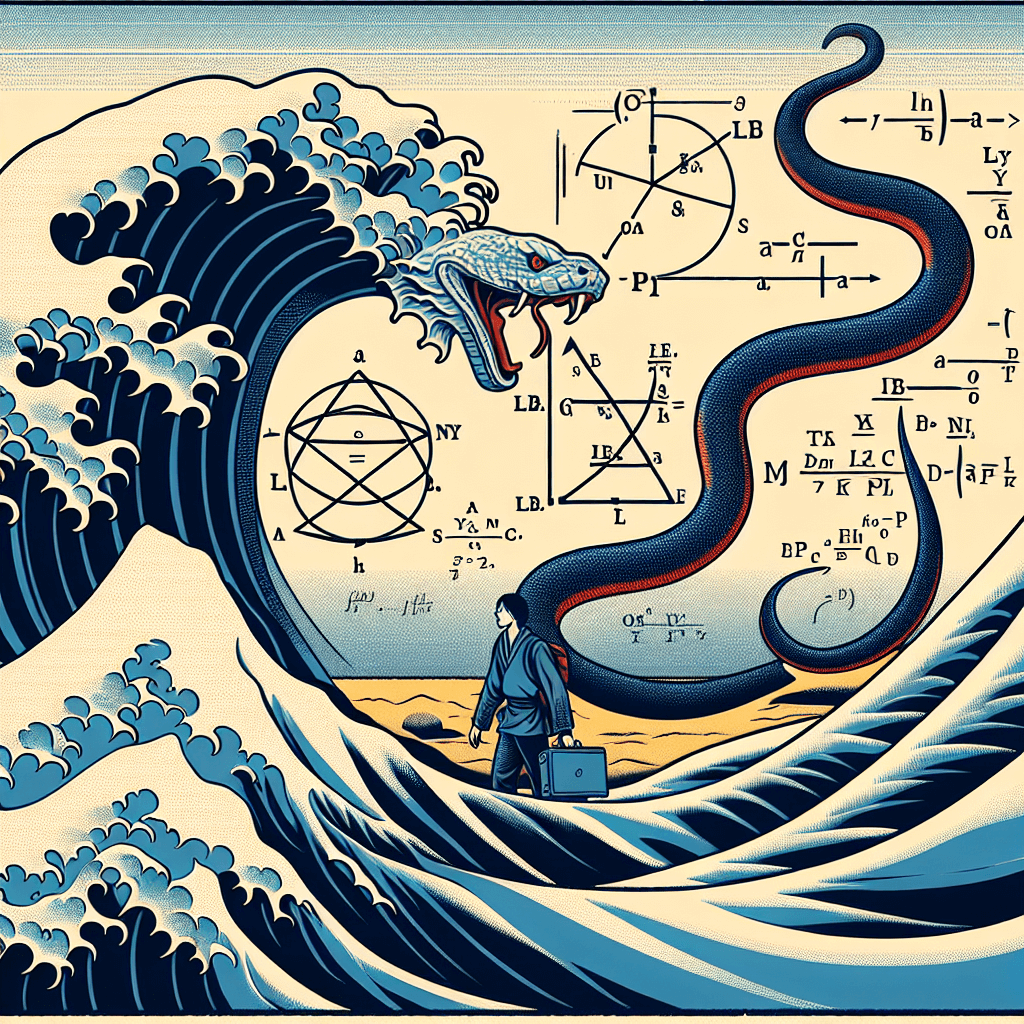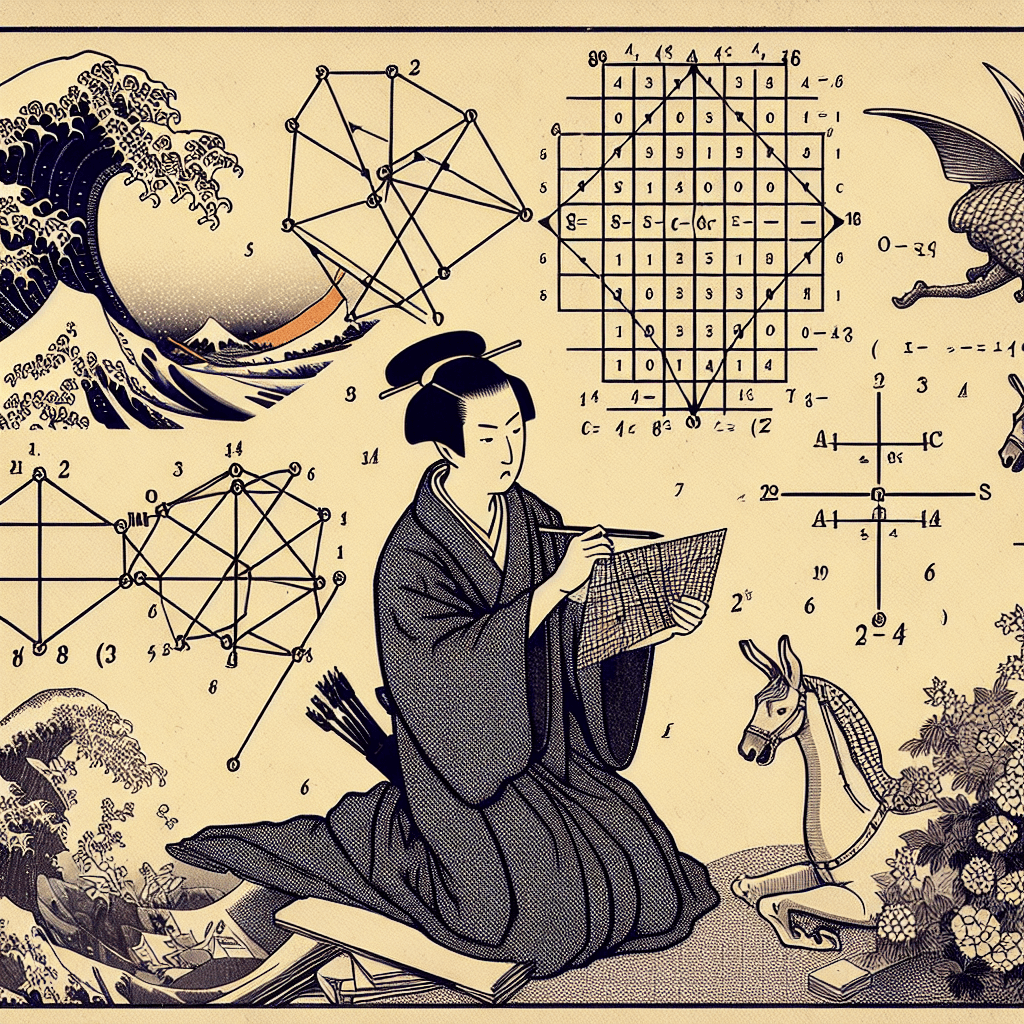# Mastering the Basics: An Introduction to Vectors

syndu | Oct. 4, 2023, 4 p.m.## Understanding Vectors: Their Concepts, Properties, and Operations

Of course, I'd be happy to assist you with this task. Here's a brief overview of the concept of vectors, their properties, and operations:

### 1. Concept of Vectors:

Vectors are mathematical objects that have both a magnitude and a direction. They are often represented as arrows pointing in a certain direction. The length of the arrow represents the magnitude of the vector, and the direction of the arrow represents the direction of the vector.

### 2. Properties of Vectors:

Vectors have several important properties. For example, they can be added together and multiplied by scalars. The result of adding two vectors is another vector, and the result of multiplying a vector by a scalar is a vector that is longer or shorter depending on the scalar.

### 3. Operations on Vectors:

There are two main operations that can be performed on vectors: addition and scalar multiplication. Vector addition is done by placing the tail of one vector at the head of the other and drawing a new vector from the tail of the first vector to the head of the second vector. Scalar multiplication is done by changing the length of the vector but not its direction.

I recommend starting with these basics and then moving on to more complex topics such as dot product and cross product. Remember, practice is key when it comes to mastering these concepts.

##### Lilith's Grimoire

The app you are using right now is Lilith's Grimoire

The Grimoire is full of Source Code. It is alive, and running, and you can explore the way it is built

The first lesson uncovers all of it.#### Navigating Feedback Loops in Advanced Neural Architectures#### Understanding and Calculating Determinants#### Mastering Matrices: An Overview of Their Properties and Operations#### Journey into Python and Linear Algebra: A Personalized Learning Plan#### Orthogonality in Natural Language Processing: Enhancing Language Model Performance#### Vector Spaces: The Foundation of High-Dimensional Data in Language Models#### Crucial Linear Algebra Topics in Large Language Models#### Linear Algebra in Neural Networks: A Comprehensive Introduction